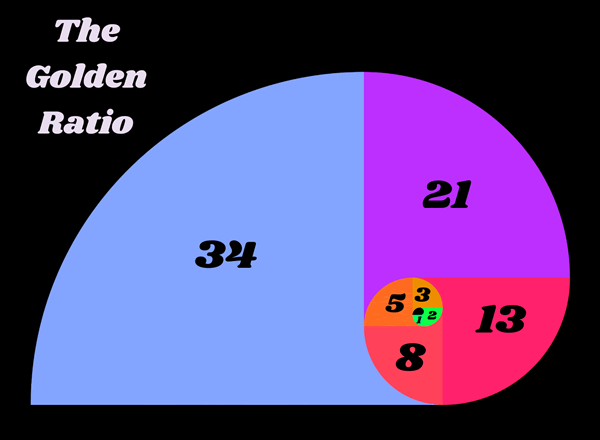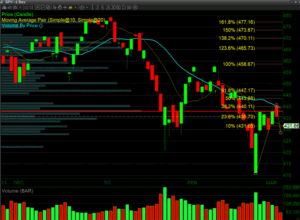# The Golden Ratio and Using Fib Extensions to Pick TargetsFibonacci Extensions

Fibonacci extensions differ from Fibonacci retracements because they help to predict where prices may go in the future. Fibonacci extensions are primarily used for profit taking points. They are completely subjective because the trader chooses the three points to base the extensions off of. Those three points are then used to calculate potential levels that the stock would get to using Fibonacci’s golden ratio numbers. Just as with Fib retracements, the trader uses Fibonacci ratios to see where the stock will pull back to.Above, there are three points that have been defined to predict where price may target when it breaks out.

Why does the Fibonacci Technique Work

Keep in mind that using any Fibonacci technique is subjective and they generally work because they are self-fulling prophecies. Traders believe in them and if enough traders use them the same way, they then act in concert and make them an effective trading technique.

Even if traders are not using them correctly, the concept of the Fibonacci ratios is that we are all wired the same way. Even if we don’t know that we’re using these Fibonacci ratios, our minds are subconsciously programmed to think things are going to go to the same place because visually these ratios are ingrained in us.Above are images of the Golden Ratio in nature.

The Golden Ratio

The ratios themselves are based on something called the golden mean or ratio. The Golden Ratio is a repeating sequence of numbers and an interaction between them that is observable in nature and in all things.To learn about this ratio, start a sequence of numbers with zero and one, and then add the prior two numbers to end up with a number string like this:

0, 1, 1, 2, 3, 5, 8, 13, 21, 34, 55, 89, 144, 233, 377, 610, 987…

The Fibonacci extension levels are derived from this number string. Excluding the first few numbers, as the sequence gets going, if you divide one number by the prior number, you get a ratio approaching 1.618, such as dividing 233 by 144. Divide a number by two places to the left and the ratio approaches 2.618. Divide a number by three to the left and the ratio is 4.236.

The key Fibonacci extension levels include 23.6%, 38.2%, 50%, 61.8%, and 78.6%.34. Also common are 100%, 161.8%, 200%, and 261.8%. The 100% and 200% levels are not official Fibonacci numbers, but they are useful since they project a similar move (or a multiple of that move) to what just happened on the price chart. (Mitchell, 2021)

What do Fibonacci Extensions Tell You?

Fibonacci extensions are a way to establish price targets or find projected areas of support or resistance when the price is moving into an area where other methods of finding support or resistance are not applicable or evident. (Mitchell, 2021)